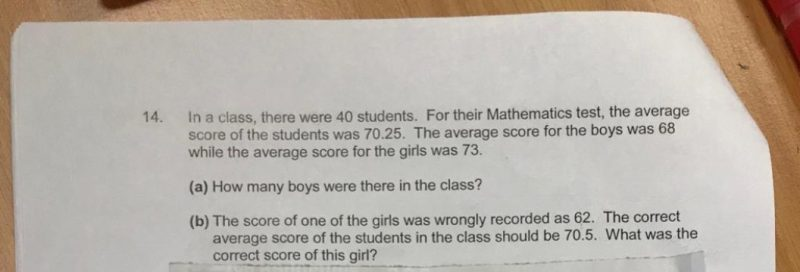# QuestionAlternative Solution without Algebra

Working 14(A):

Total Score for Class = 40 * 70.25 = 2810

Total Score if all students scored 68 = 40 * 68 = 2720

Total Score difference girls made = 2810 – 2720 = 90

Difference btw girls average and boys average score = 73 – 68 = 5 points

Number of girls = 90 / 5 = 18

Number of boys = 40 – 18 = 22.

0 Replies 0 Likes

Working for 14(A):

Total Score of 40 Students = 40 * 70.25 = 2810

Average Score for Boys = 68 * X. Let X be number of Boys.

Average Score for Girls = 73 * Y. Let Y be number of Girls.

Equation 1:
X + Y = 40, Y = 40 – X

Equation 2:
68X + 73Y = 2810

Combining Equation 1 and Equation 2:
68X + 73(40-X) = 2810
68X + 2920 – 73X = 2810
5X = 110
X = 22

Answer 14(A): There are 22 Boys.

———————–

Working for 14(B):

Total Score = 40 * 70.5 = 2820

Difference before and after = 2820 – 2810 = 10 points difference

Girl’s correct score is = 62 +10 = 72.

Answer 14(B): Girl’s correct score is 72.

0 Replies 0 Likes